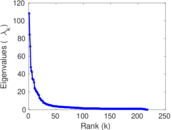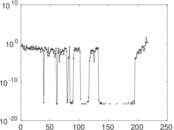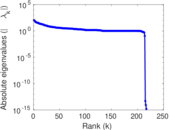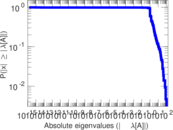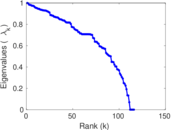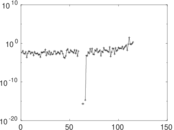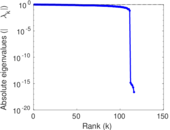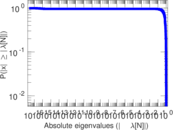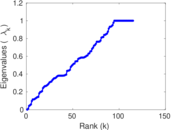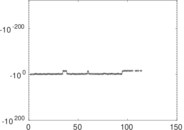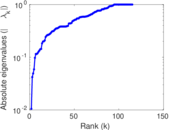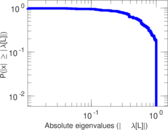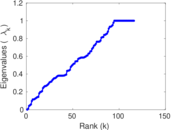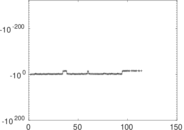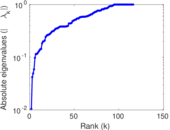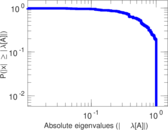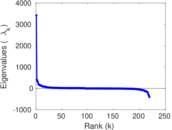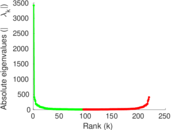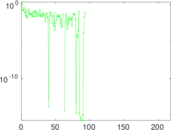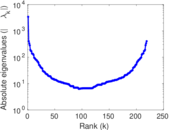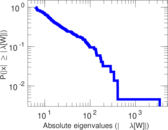# Wikibooks edits (si)

This is the bipartite edit network of the Sinhala Wikibooks. It contains users and pages from the Sinhala Wikibooks, connected by edit events. Each edge represents an edit. The dataset includes the timestamp of each edit.

 Code `bsi` Internal name `edit-siwikibooks` Name Wikibooks edits (si) Data source http://dumps.wikimedia.org/ AvailabilityDataset is available for download Consistency checkDataset passed all tests Category Authorship network Dataset timestamp 2017-10-20 Node meaning User, article Edge meaning Edit Network formatBipartite, undirected Edge typeUnweighted, multiple edges Temporal dataEdges are annotated with timestamps

## Statistics

 Size n = 3,427 Left size n1 = 219 Right size n2 = 3,208 Volume m = 5,850 Unique edge count m̿ = 3,288 Wedge count s = 1,039,610 Claw count z = 368,713,927 Cross count x = 105,725,355,173 Square count q = 5,599 4-Tour count T4 = 4,209,872 Maximum degree dmax = 1,922 Maximum left degree d1max = 1,922 Maximum right degree d2max = 100 Average degree d = 3.414 06 Average left degree d1 = 26.712 3 Average right degree d2 = 1.823 57 Fill p = 0.004 680 08 Average edge multiplicity m̃ = 1.779 20 Size of LCC N = 2,666 Diameter δ = 13 50-Percentile effective diameter δ0.5 = 3.481 21 90-Percentile effective diameter δ0.9 = 5.704 72 Median distance δM = 4 Mean distance δm = 4.018 98 Gini coefficient G = 0.704 927 Balanced inequality ratio P = 0.219 060 Left balanced inequality ratio P1 = 0.106 154 Right balanced inequality ratio P2 = 0.323 077 Relative edge distribution entropy Her = 0.746 968 Power law exponent γ = 7.389 44 Tail power law exponent γt = 3.051 00 Tail power law exponent with p γ3 = 3.051 00 p-value p = 0.001 000 00 Left tail power law exponent with p γ3,1 = 1.771 00 Left p-value p1 = 0.557 000 Right tail power law exponent with p γ3,2 = 3.471 00 Right p-value p2 = 0.000 00 Degree assortativity ρ = −0.277 010 Degree assortativity p-value pρ = 5.385 55 × 10−59 Spectral norm α = 108.246 Algebraic connectivity a = 0.010 500 3 Spectral separation |λ1[A] / λ2[A]| = 1.282 75 Controllability C = 2,591 Relative controllability Cr = 0.858 231

## Plots

### Fruchterman–Reingold graph drawing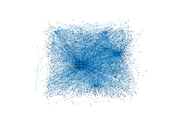### Degree distribution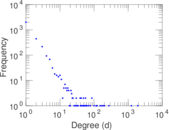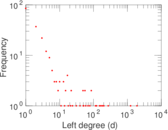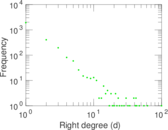### Cumulative degree distribution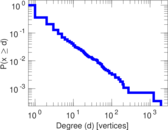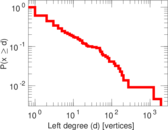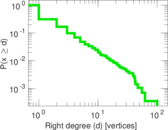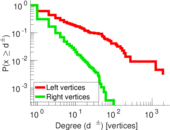### Lorenz curve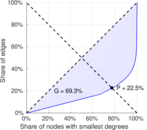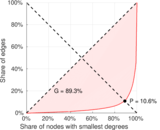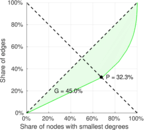### Spectral distribution of the adjacency matrix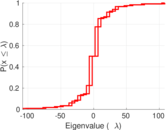### Spectral distribution of the normalized adjacency matrix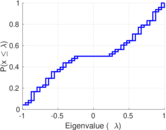### Spectral distribution of the Laplacian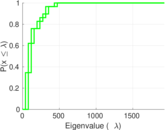### Spectral graph drawing based on the adjacency matrix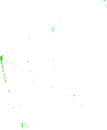### Spectral graph drawing based on the Laplacian### Spectral graph drawing based on the normalized adjacency matrix### Degree assortativity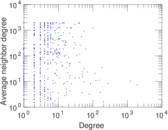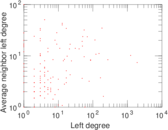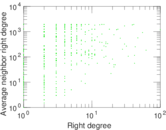### Zipf plot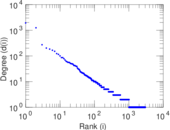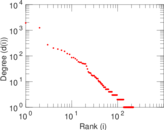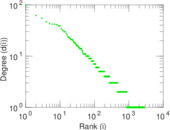### Hop distribution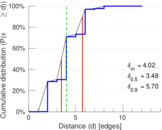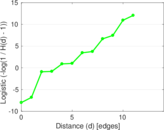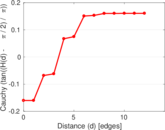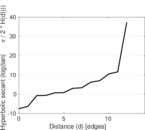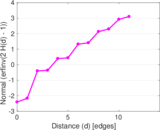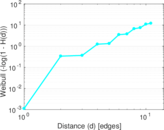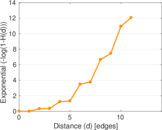### Double Laplacian graph drawing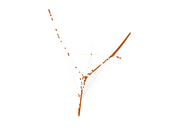### Delaunay graph drawing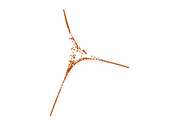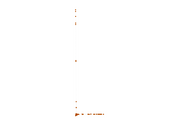### Edge weight/multiplicity distribution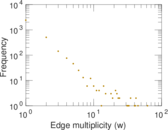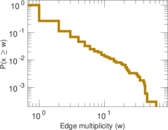### Temporal distribution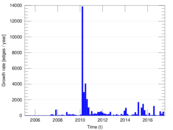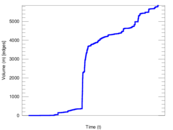### Temporal hop distribution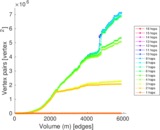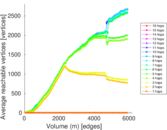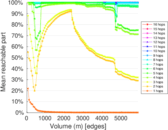### Diameter/density evolution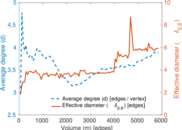### Matrix decompositions plots